# RD Sharma Solutions Class 12 Probability Exercise 31.1

RD Sharma Solutions for Class 12 Maths Chapter 31 Probability Exercise 31.1 are provided here. Subject experts at BYJU’S have curated these solutions in a very lucid manner so that it helps all students to solve problems in the right way. Students who wish to achieve their desired score in the subject must practise the RD Sharma Class 12 Solutions regularly. The PDF containing solutions to this exercise can be downloaded effortlessly from the link provided below and can be used for future reference at any time.

## Download PDF of RD Sharma Solutions for Class 12 Maths Chapter 31 Exercise 1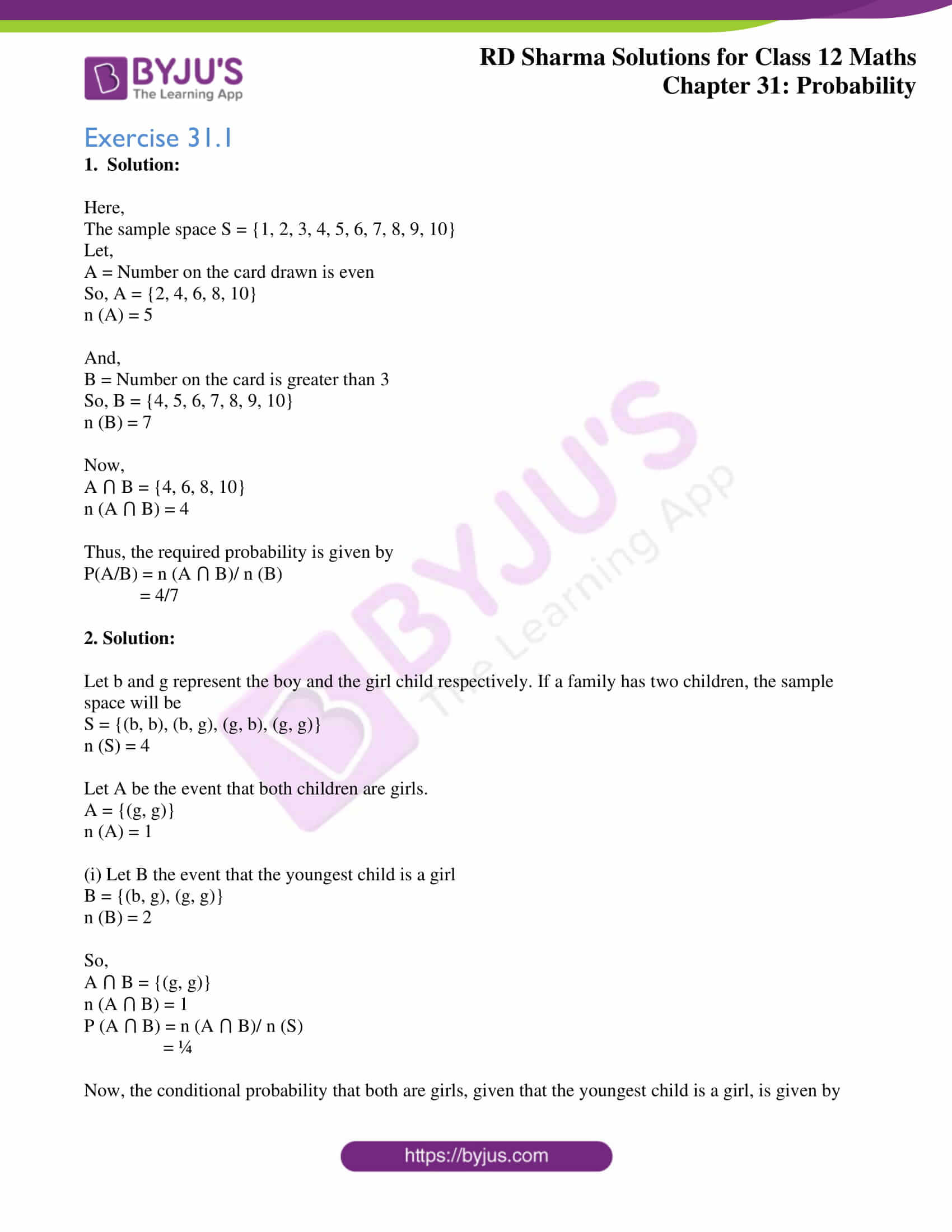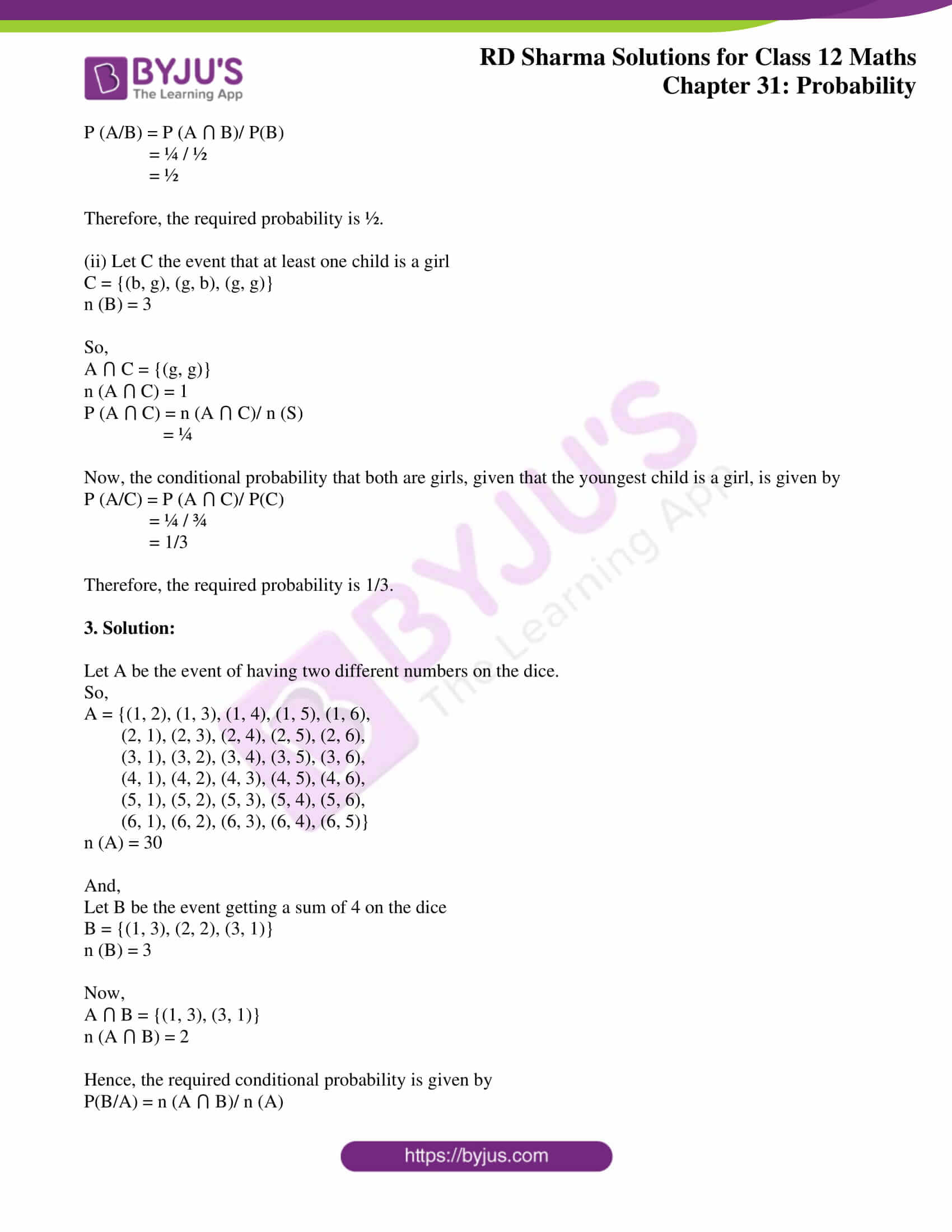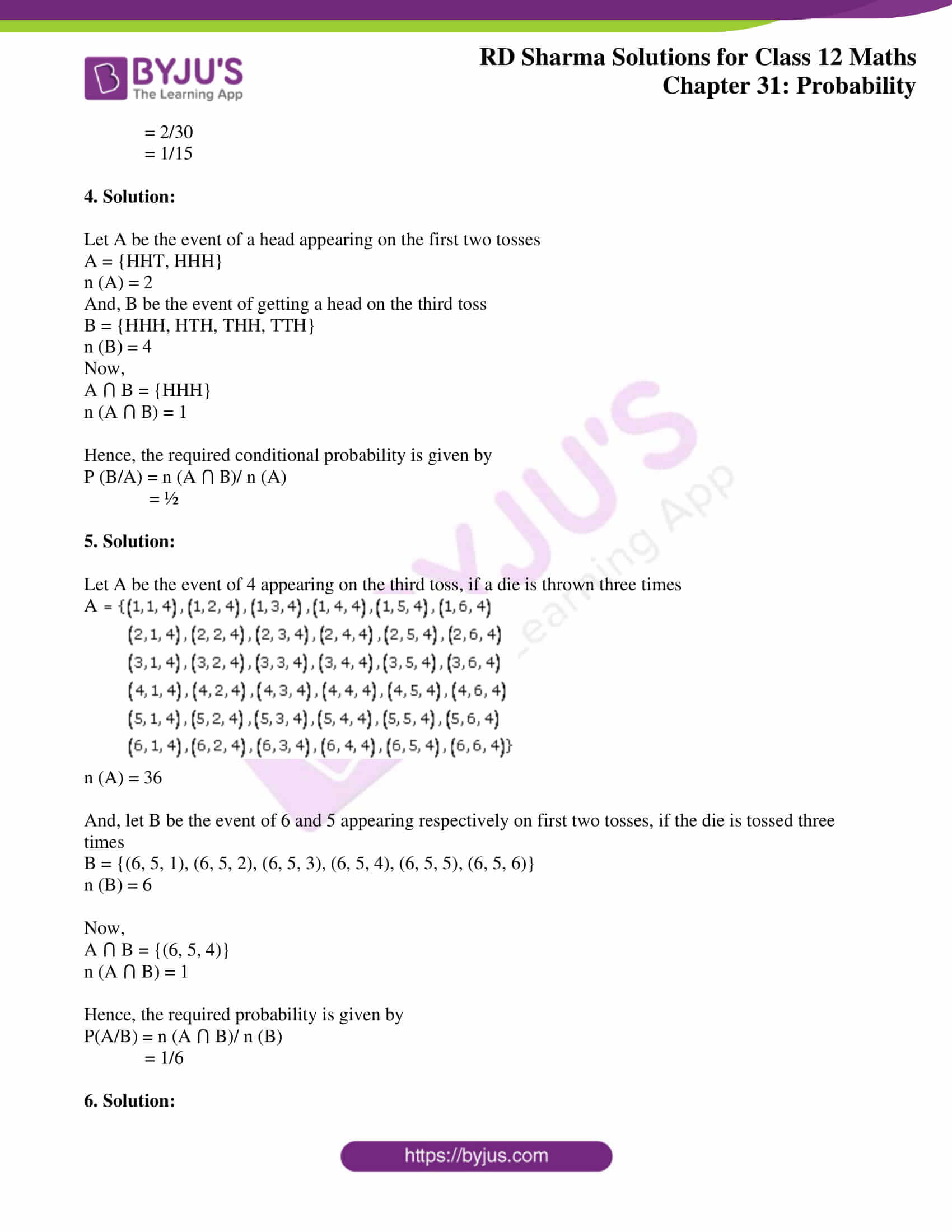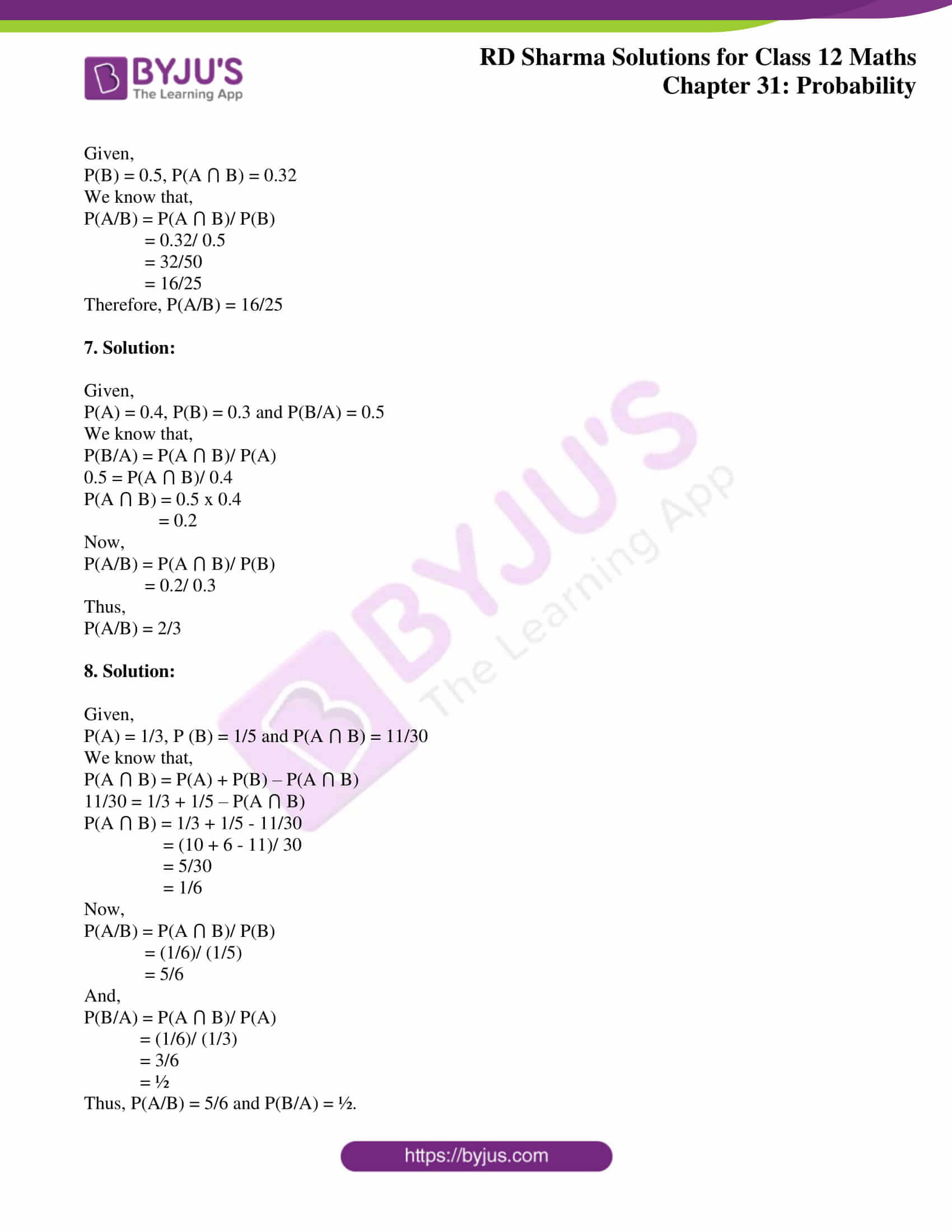### Access RD Sharma Solutions for Class 12 Maths Chapter 31 Exercise 1

Exercise 31.1

1. Solution:

Here,

The sample space S = {1, 2, 3, 4, 5, 6, 7, 8, 9, 10}

Let,

A = Number on the card drawn is even

So, A = {2, 4, 6, 8, 10}

n (A) = 5

And,

B = Number on the card is greater than 3

So, B = {4, 5, 6, 7, 8, 9, 10}

n (B) = 7

Now,

A ⋂ B = {4, 6, 8, 10}

n (A ⋂ B) = 4

Thus, the required probability is given by

P(A/B) = n (A ⋂ B)/ n (B)

= 4/7

2. Solution:

Let b and g represent the boy and the girl child respectively. If a family has two children, the sample space will be

S = {(b, b), (b, g), (g, b), (g, g)}

n (S) = 4

Let A be the event that both children are girls.

A = {(g, g)}

n (A) = 1

(i) Let B the event that the youngest child is a girl

B = {(b, g), (g, g)}

n (B) = 2

So,

A ⋂ B = {(g, g)}

n (A ⋂ B) = 1

P (A ⋂ B) = n (A ⋂ B)/ n (S)

= ¼

Now, the conditional probability that both are girls, given that the youngest child is a girl, is given by

P (A/B) = P (A ⋂ B)/ P(B)

= ¼ / ½

= ½

Therefore, the required probability is ½.

(ii) Let C the event that at least one child is a girl

C = {(b, g), (g, b), (g, g)}

n (B) = 3

So,

A ⋂ C = {(g, g)}

n (A ⋂ C) = 1

P (A ⋂ C) = n (A ⋂ C)/ n (S)

= ¼

Now, the conditional probability that both are girls, given that the youngest child is a girl, is given by

P (A/C) = P (A ⋂ C)/ P(C)

= ¼ / ¾

= 1/3

Therefore, the required probability is 1/3.

3. Solution:

Let A be the event of having two different numbers on the dice.

So,

A = {(1, 2), (1, 3), (1, 4), (1, 5), (1, 6),

(2, 1), (2, 3), (2, 4), (2, 5), (2, 6),

(3, 1), (3, 2), (3, 4), (3, 5), (3, 6),

(4, 1), (4, 2), (4, 3), (4, 5), (4, 6),

(5, 1), (5, 2), (5, 3), (5, 4), (5, 6),

(6, 1), (6, 2), (6, 3), (6, 4), (6, 5)}

n (A) = 30

And,

Let B be the event getting a sum of 4 on the dice

B = {(1, 3), (2, 2), (3, 1)}

n (B) = 3

Now,

A ⋂ B = {(1, 3), (3, 1)}

n (A ⋂ B) = 2

Hence, the required conditional probability is given by

P(B/A) = n (A ⋂ B)/ n (A)

= 2/30

= 1/15

4. Solution:

Let A be the event of a head appearing on the first two tosses

A = {HHT, HHH}

n (A) = 2

And, B be the event of getting a head on the third toss

B = {HHH, HTH, THH, TTH}

n (B) = 4

Now,

A ⋂ B = {HHH}

n (A ⋂ B) = 1

Hence, the required conditional probability is given by

P (B/A) = n (A ⋂ B)/ n (A)

= ½

5. Solution:

Let A be the event of 4 appearing on the third toss, if a die is thrown three times

A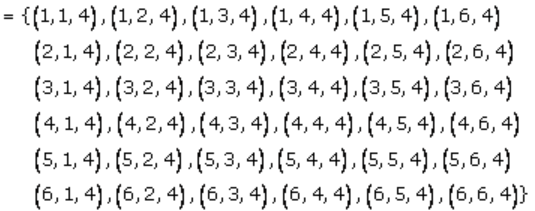n (A) = 36

And, let B be the event of 6 and 5 appearing respectively on first two tosses, if the die is tossed three times

B = {(6, 5, 1), (6, 5, 2), (6, 5, 3), (6, 5, 4), (6, 5, 5), (6, 5, 6)}

n (B) = 6

Now,

A ⋂ B = {(6, 5, 4)}

n (A ⋂ B) = 1

Hence, the required probability is given by

P(A/B) = n (A ⋂ B)/ n (B)

= 1/6

6. Solution:

Given,

P(B) = 0.5, P(A ⋂ B) = 0.32

We know that,

P(A/B) = P(A ⋂ B)/ P(B)

= 0.32/ 0.5

= 32/50

= 16/25

Therefore, P(A/B) = 16/25

7. Solution:

Given,

P(A) = 0.4, P(B) = 0.3 and P(B/A) = 0.5

We know that,

P(B/A) = P(A ⋂ B)/ P(A)

0.5 = P(A ⋂ B)/ 0.4

P(A ⋂ B) = 0.5 x 0.4

= 0.2

Now,

P(A/B) = P(A ⋂ B)/ P(B)

= 0.2/ 0.3

Thus,

P(A/B) = 2/3

8. Solution:

Given,

P(A) = 1/3, P (B) = 1/5 and P(A ⋂ B) = 11/30

We know that,

P(A ⋂ B) = P(A) + P(B) – P(A ⋂ B)

11/30 = 1/3 + 1/5 – P(A ⋂ B)

P(A ⋂ B) = 1/3 + 1/5 – 11/30

= (10 + 6 – 11)/ 30

= 5/30

= 1/6

Now,

P(A/B) = P(A ⋂ B)/ P(B)

= (1/6)/ (1/5)

= 5/6

And,

P(B/A) = P(A ⋂ B)/ P(A)

= (1/6)/ (1/3)

= 3/6

= ½

Thus, P(A/B) = 5/6 and P(B/A) = ½.